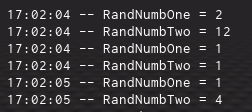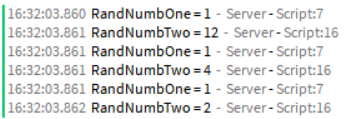# Random Number gen keeps repeating

I dont know if my brain is just fried or what

But When this function runs the first loop is actually random.
but then the other 3 times keeps giving repeated

``````function GetRandom()
for i = 1,3 do
RandomNumber = math.random(1,2)
print("RandNumbOne = ".. tonumber(RandomNumber))
RandomNumber = math.random(1,12)
print("RandNumbTwo = ".. tonumber(RandomNumber))
end
end
GetRandom()

``````

Output: (etc)
RandNumbOne =2
RandNumbTwo = 4
RandNumbOne =1
RandNumbTwo = 12
RandNumbOne =1
RandNumbTwo = 12

this could possibly because you didnt make the variable change and it’s repeating the same thing

edit: you made it do that 3 times right? every time it’s gonna print the same numbers

Try something with `math.randomseed`, the function shouldn’t be repeating the same numbers every time after I ran some analysis.

Would you consider adding a break into the `for` loop?

``````function GetRandom()
for i = 1,3 do
RandomNumber = math.random(1,2)
print("RandNumbOne = ".. tonumber(RandomNumber))
RandomNumber = math.random(1,12)
print("RandNumbTwo = ".. tonumber(RandomNumber))
break
end
end
GetRandom()
``````

ive tried declaring the variable before the function, Ive made it so when i declare it inside the function its local, i just dont know

I tried setting the for loop to i = 1,100
and it keeps repeating the pattern

That’s not my suggestion, use `math.randomseed` function which is different from `math.random`, it sets a seed.

This code works fine for me:

``````math.randomseed(os.time())

function GetRandom()
for i = 1, 3 do
local RandomNumberOne = math.random(1, 2)
print("RandNumbOne = " .. tostring(RandomNumberOne))
local RandomNumberTwo = math.random(1, 12)
print("RandNumbTwo = " .. tostring(RandomNumberTwo))
end
end

GetRandom()
``````
2 Likes

to make it simple i just added a variable and it prints like this``````function GetRandom()
local RandomNumber
for i = 1,3 do
RandomNumber = math.random(1,2)
print("RandNumbOne = ".. tonumber(RandomNumber))
RandomNumber = math.random(1,12)
print("RandNumbTwo = ".. tonumber(RandomNumber))
end
end
GetRandom()``````

That’s because you’re not localizing your variables, causing the loops that are running before it change the current loops globals, use @jmt99’s example:

Although you don’t really need to use randomseed.

1 Like

its because i was trying loads off different stuff to fix it so i tried un-localising them, and then gave up and just posted the code on here.

i have tried localising them and making the variables for (1,2) and (1,12) different names but to no avail

Maybe its cause i over simplified it, its not a exact copy of the code cause i didnt want it to confuse anyone

heres the actual code

``````function Raid()

--Creating Raiders

for i = 1,3 do

local RandomNumber = math.random(1,2)
local Gender = nil

if RandomNumber == 1 then
Gender = "Male"
else
Gender = "Female"
end

Raider = game.Lighting:FindFirstChild(Gender):Clone()
Raider.Parent = game.Workspace.Raiders
Raider:MoveTo(game.Workspace.SpawnPoint.Position)
Raider.Name = "Raider"

local RandomNumberTwo = math.random(1,12)
local Hair = game.Lighting.Hair:FindFirstChild(Gender.. "-".. tostring(RandomNumberTwo)):Clone()

end

end

Raid()
``````

(Edit: i did put prints inside it and they were just being repeated like the example at the top. so i know its the number generator and not the important lines)

Still works fine for me.

Modified script
``````function Raid()

--Creating Raiders

for i = 1,3 do

local RandomNumber = math.random(1,2) print("RandNumbOne = " .. RandomNumber)
local Gender = nil

if RandomNumber == 1 then
Gender = "Male"
else
Gender = "Female"
end

local RandomNumberTwo = math.random(1,12) print("RandNumbTwo = " .. RandomNumberTwo)
--local Hair = game.Lighting.Hair:FindFirstChild(Gender.. "-".. tostring(RandomNumberTwo)):Clone()

end

end

Raid()
``````

Output:Maybe try using a repeat loop instead of a for loop

``````function Raid()
local tries = 0
--Creating Raiders
repeat
local RandomNumber = math.random(1,2)
local Gender = nil

if RandomNumber == 1 then
Gender = "Male"
else
Gender = "Female"
end

local RandomNumberTwo = math.random(1,12)
local Hair = game.Lighting.Hair:FindFirstChild(Gender.. "-".. tostring(RandomNumberTwo)):Clone()

tries += 1
until tries == 3
end

Raid()
``````

`math.random` has a tendency to do that. However, you can use Random | Roblox Creator Documentation instead. Random doesn’t have the problem if repeating numbers.

In your code specifically, you would create a Random object using `Random.new` and call either `Random:NextNumber` or ` Random:NextInteger` in place of `math.random`.

Sorry there was another part i didnt include because i didnt think it was needed, But i think its the thing thats breaking it

Ive edited my previous reply so now its the full code, nothing left out

Have you tried putting break at the end of the loop?

No, because whats the point of the loop if it only runs once?

1 Like

using `break` will break the loop (as its name). We don’t want to break the loop

2 Likes